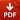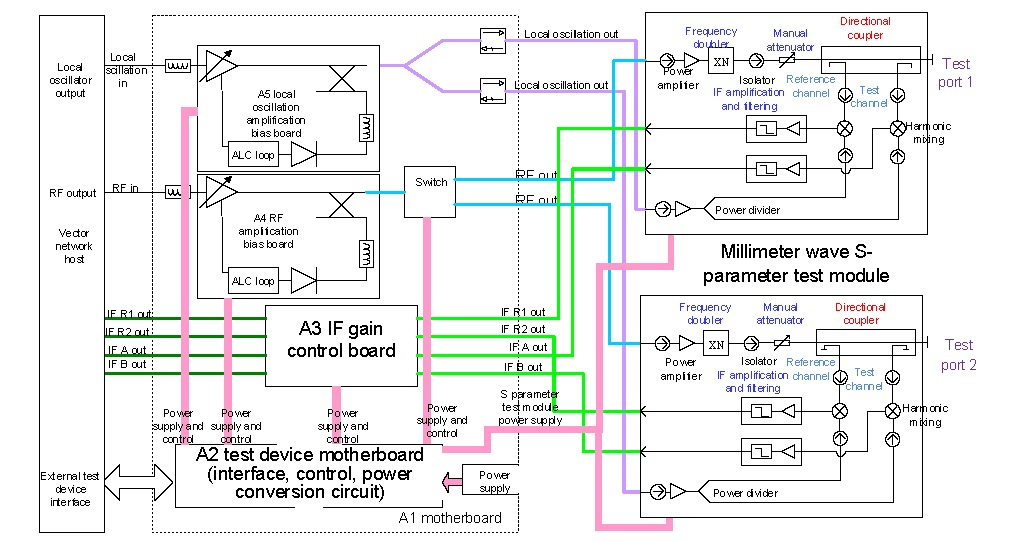## Millimeter wave (THz) VNA System Solution (50 GHz ~ 500 GHz)

Select Options

The millimeter-wave (THz) vector network analyzer adopts split-type structure and the structural system which uses GPIB bus as the system control bus.THz Test Modules – Selection-GuideTHz Test Modules

## Millimeter wave (THz) VNA System Solution (50 GHz ~ 500 GHz)

### System solution

3.1 Millimeter wave (THz) Vector Network Analyzer

The millimeter-wave (THz) vector network analyzer adopts split-type structure and the structural system which uses GPIB bus as the system control bus. By changing the S-parameter test module in different frequency bands, measurement of network parameters in different frequency bands can be completed.

(1) System working principle

The principle block diagram of the vector network analysis system is shown in Figure 1.Figure 1 Block diagram of the vector network analysis system

(2) The core of the vector network analysis system is the controller and the millimeter wave (THz) S parameter test module. In the controller, the RF signal from the vector network is amplified by the microwave amplitude stabilization amplification component. The PIN electronic switch is a key component for realizing forward and reverse S-parameter tests. When the forward S-parameter test is performed, the microwave excitation signal is output into the millimeter wave (THz) S-parameter test module of Port 1, and the RF excitation signal drives the power amplifier and frequency doubler in the millimeter wave (THz) S-parameter test module to generate the millimeter wave (THz) excitation signal. The excitation signal of millimeter wave (THz) is applied to the network under test through the main path of the two cascade directional couplers in the probe. The signal representing the incident wave of the device under test is taken out of the coupling port of the first directional coupler and denoted by R1, and the reflected signal of the device under test is taken out of the coupling port of the second directional coupler and denoted by A. The transmitted signal of the DUT is taken out through the coupling port of the directional coupler of the S-parameter test module of port 2 and denoted by B. The local oscillation signal from the vector network local oscillation output is divided into four local oscillation signals in the controller by the power divider and then amplified by the local oscillation amplifier before being sent to the harmonic mixer. The millimeter wave (THz) incident wave R1, the reflected wave A, and the transmitted wave B, which represent the characteristics of the device under test are mixed with the harmonics of the local oscillation signal and converted into a 7.605634 MHz IF signal. The IF output by the harmonic mixer is amplified by the controller and sent to the host computer for processing, in this manner to obtain
the forward S parameter of the device under test: S11=R1/A, S21=B/R1; similarly, the inverse S-parameters of the network under test can be obtained as well: S12 = A/R2, S22 = B/R2. The test results can be read, print and stored by the main control computer.